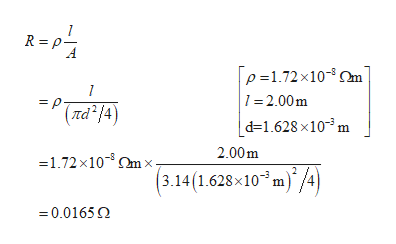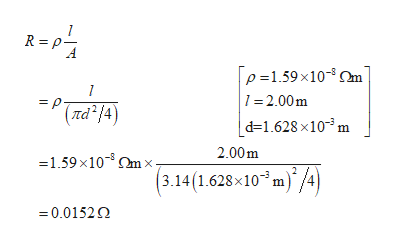# 25.13     A 14-gauge copper wire of diameter 1.628 mm carries a current of 12.5 mA. (a) What is the potential difference across a 2.00-m length of the wire? (b) What would the potential difference in part (a) be if the wire were silver instead of copper, but all else were the same?HINT: It is not necessary to work this problem again for part (b).

Question
44 views

25.13     A 14-gauge copper wire of diameter 1.628 mm carries a current of 12.5 mA. (a) What is the potential difference across a 2.00-m length of the wire? (b) What would the potential difference in part (a) be if the wire were silver instead of copper, but all else were the same?

HINT: It is not necessary to work this problem again for part (b).

check_circle

Step 1

The diameter of the wire is 1.628mm, and current through the copper wire is 12.5mA, and the resistivity of copper wire is 1.72x10-8ohm meter.

(a)Write the expression for the resistance of the wire.help_outlineImage TranscriptioncloseR p p 1.72x10-m 1 2.00m 1 = p. (Itd 14) | d=1.628 x103 m 2.00m =1.72x10mx (3.14(1.628x10 m/4 0.0165 Q fullscreen
Step 2

Write the expression for the potential energy.

Step 3

(b)The resistivity of silver is 1.59x10-8ohm meter.

Write the...help_outlineImage TranscriptioncloseR =pτ p 1.59x10-m 1 -2.00m 1 = p. (rd 4) | d=1.628 x103 m 2.00m -1.59x10%mx (3.14(1.628x10 m/4) =0.01520 fullscreen

### Want to see the full answer?

See Solution

#### Want to see this answer and more?

Solutions are written by subject experts who are available 24/7. Questions are typically answered within 1 hour.*

See Solution
*Response times may vary by subject and question.
Tagged in

### Current Electricity